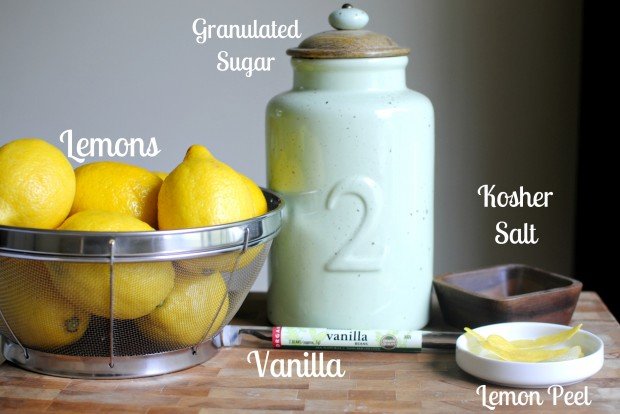# Matrix Multiplication in Real-Life

Hello World,

Before discussing about matrix multiplication, let me start this discussion with even more basic question. What does simple multiplication means?

To me, it feel like this. Say your IQ is 120. You have a magic wand, you wave it and say “Let my IQ go twice”. With multiplication you can grow or shrink some quantity as you wish.

Suppose I am having a Rs. 1000/- note in my purse and if  I wish that “Let the amount in purse  go 1.5 x” will turn the Rs. 1000/- to Rs. 1500/-   :-)))

We can’t multiply things that don’t exist. I don’t have a private Jet Air plane so does I can’t multiply it.

This concept can be thought of shrinking or expanding values.

Let’s take a simple case of $1\times N$ and $N\times 1$ element matrix multiplication.

Analogy 1

$\begin{multline} \begin{pmatrix}Lemon & Sugar & Salt &Water\end{pmatrix}\times \begin{pmatrix} 2\quad Spoons\\ 2\quad Spoons\\ 0.5\quad Spoon\\ 1\quad Glass \end{pmatrix} \\= \begin{pmatrix}Lemonade\quad with\quad particular\quad taste\end{pmatrix} \end{multline}$

In this analogy, ingredients  are kept on a table as a row and recipe as column

[Ingredients][quantity] = [final product]

The output number can be thought of as the taste or quality of the final product. Although it is not a perfect analogy (eg. negative spoons are not possible in real life, – 2 spoons of sugar means nothing), the point here is we made a connection between abstract matrix multiplication and a real life event and chances are rare that we forget both together.

Analogy 2

$\begin{multline} \begin{pmatrix}quizzes&Assignments&Midterm&Final \end{pmatrix}\times \begin{pmatrix} 10\%\\ 20\%\\ 20\%\\ 50\%\\ \end{pmatrix}\\ = \begin{pmatrix}Final Mark\end{pmatrix} \end{multline}$

The instructor can adjust the weights as you want is an additional insight here!. Isn’t this process looks like the features in machine learning or weights of a neural network ?

Creative questions on this topic

1. What is the need for such a row column rule ? Easy readability..?
2. Why don’t A*B != B*A..?

Conclusion

Let me wind up from the simple matrix multiplication concept! All those who are interested in this topic , please share your valuable suggestions and of course that  will add lot of values to the topic. Matrix multiplication is related to Dot  Product,  we will explore it in another post.